BodhiAI
Nov. 11, 2019

#### Defination of Function,Domain & Range of a Function:

Function

Let A and B be two non-empty sets, then a function from set A to set B is a rule or method or correspondence. Thus if f denotes a rule of correspondence by which to every independent element x of set A, there corresponds one and only one dependent element y of the set B, then the correspondence f is said to be a function from set A to the set B.

This correspondence is denoted by y =f(x)

Important

From the definition of a function, if follows that

a) there may exist some elements in set B which may not have any corresponding element in set A.

b) also, two or more elements in set A may correspond to the same element in set B.

A correspondence which is one-to-one or many-to-one is called a function, a one-to-many or many-to-many correspondence cannot be a function.

In other words, a function f from a set A to set associates each element of set A to a unique element of set B.

The terms, "map" (or "maping"), "correspondence" are used as synonyms for "function".

Now, if f is a function from a set A to set B, then

f: AB or AB

which is read as f is a function from A to B or f maps A to B.

If an element aA is associated to an element bB, then b is called the f-image of a or image of a under f or the value of the function f at a. Also, a is called the pre-image of b under the function f.

Important

The correspondence f : AB is a function, iff

a) all the independent elements (inputs) in A must have their images in set B.

b) to each and every independent element in A there corresponds one and only one image in B.

c) every function is a relation but every relation may or may  not be a function.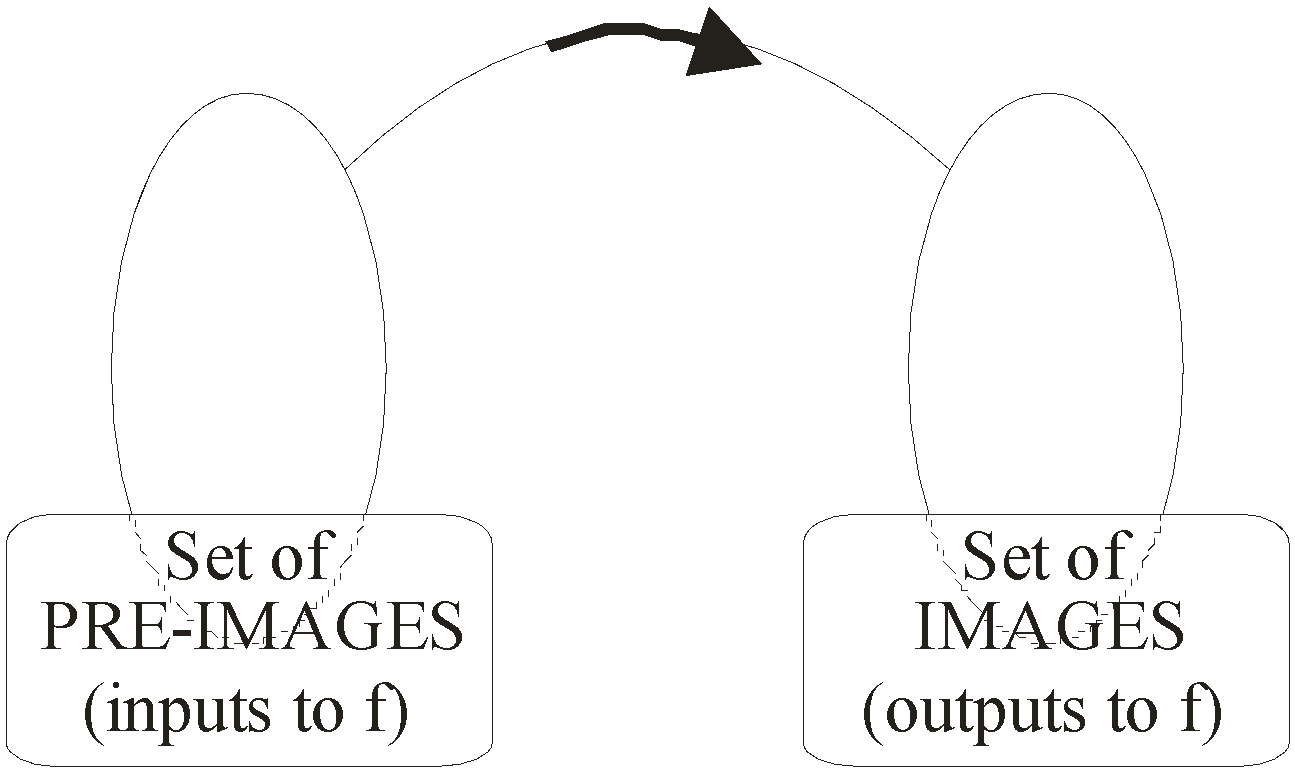Problem Solving Trick

Geometrical test for a relation to be a function : VPL Test

Geometrically, if we draw Vertical Parallel Line (VPL) i.e. any line which is parallel to y-axis (x = a), then if this line intersects the graph of the expression in more than one point, then the expression is a relation else if it intersects at only one point, the expression is a function.

Example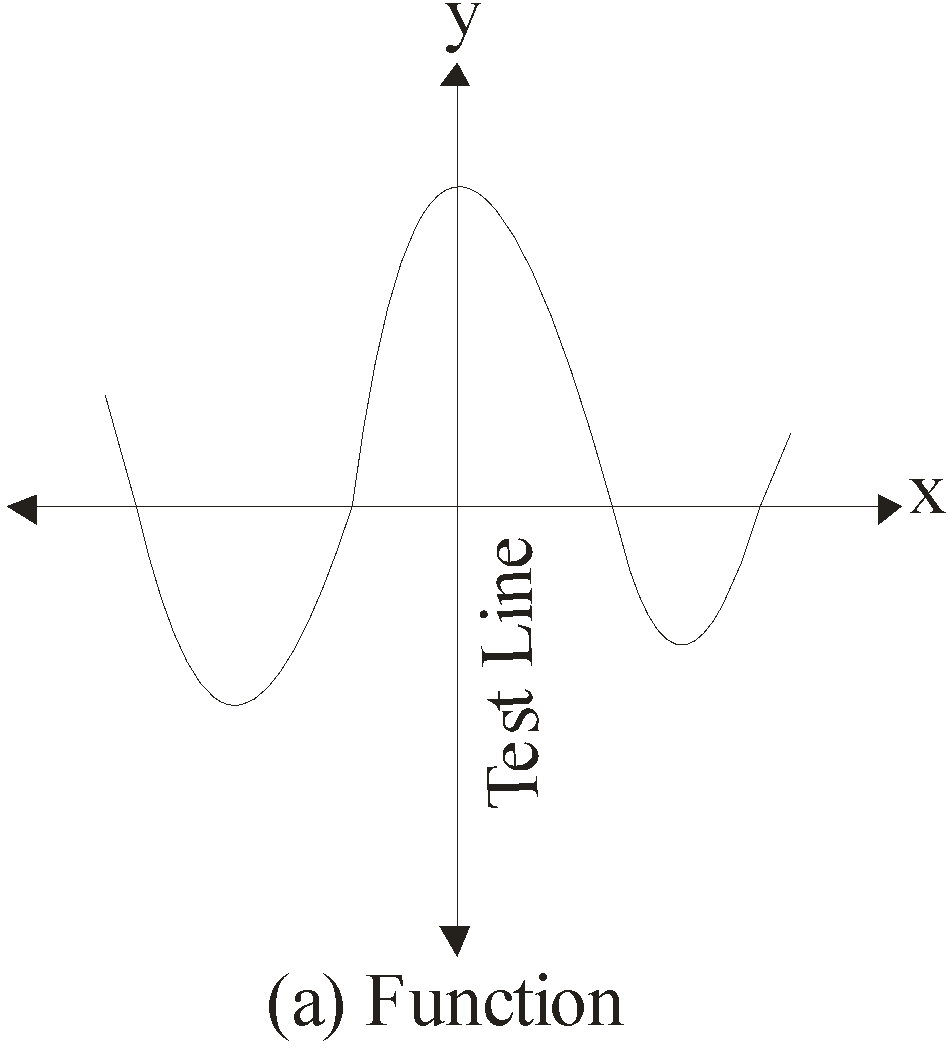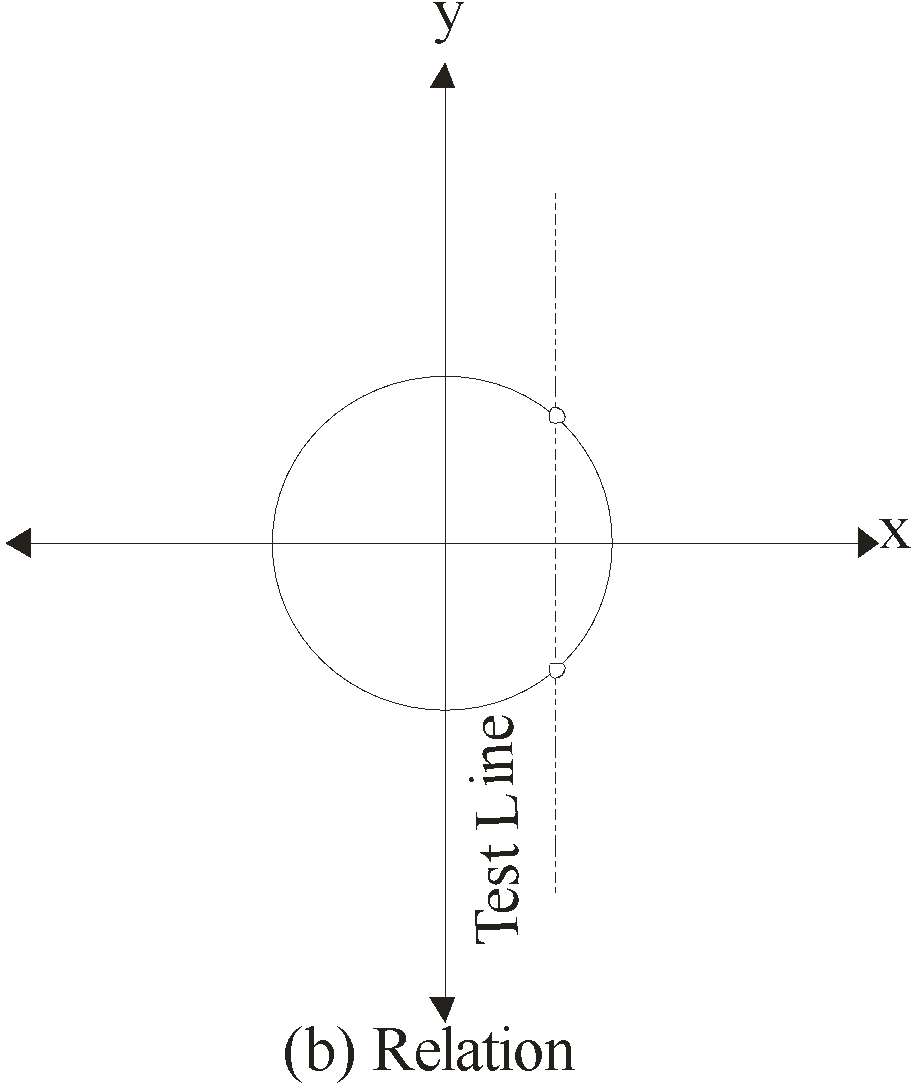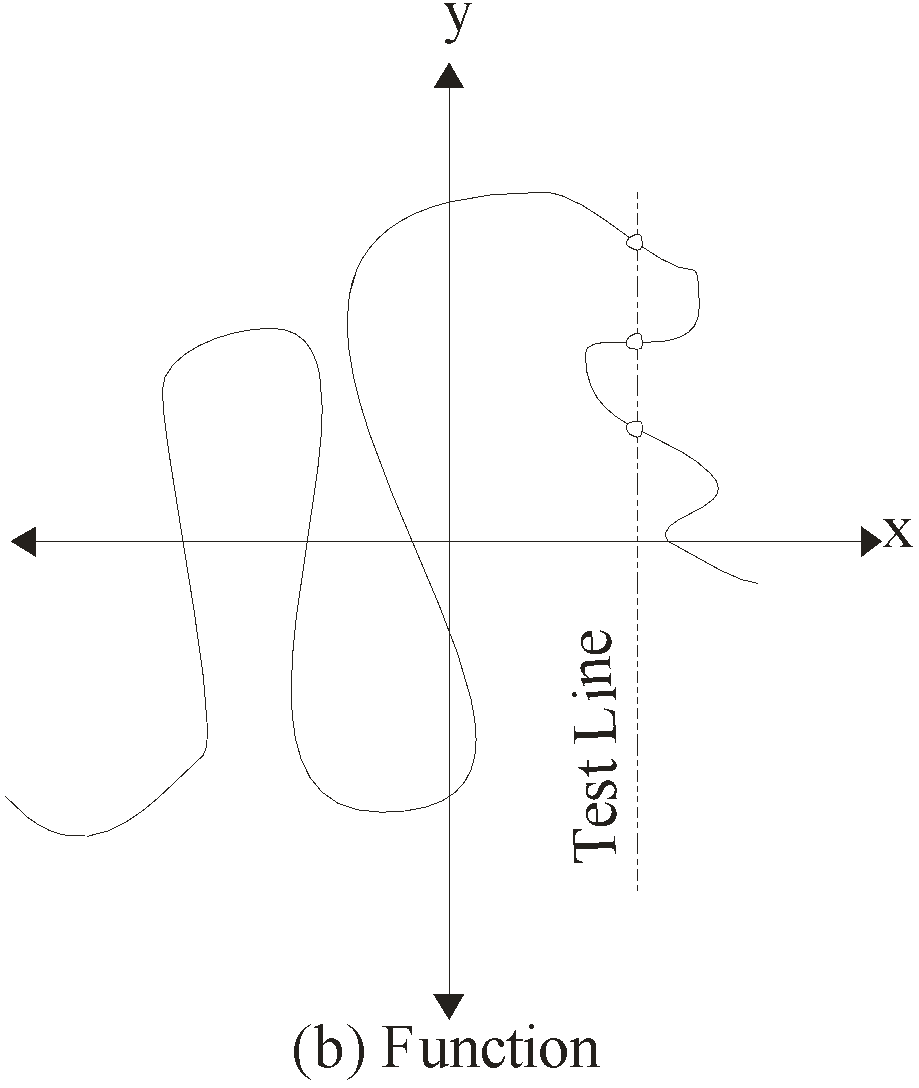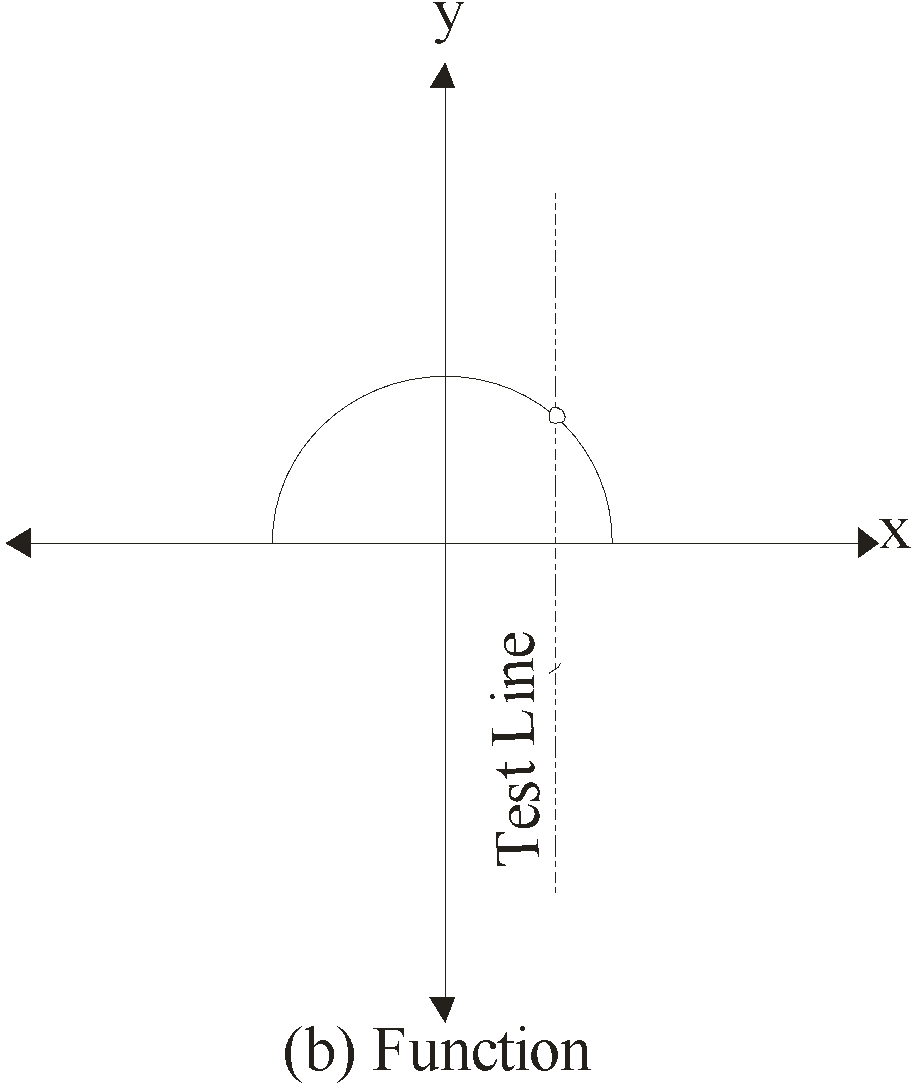Reasoning: Since we know that onle one-to-one and many-to-one correspondences represent function. Also many-to-many and one-to-many correspondeces don't represent function.This is what VPL Test checks for.

By actually drawing a Vertical Parallel line, we are actually keeping the x part same throughout for different values of y and if this line cuts or intersects the graph in more than one point, then for all different points we get all those different y for same x i.e. same values of x are giving different values of y (images) which is one-many.

Domain, Co-Domain and Range of Function

Let y = f(x) is a function, such that f : AB

Graphically, we can represent the function f as shown below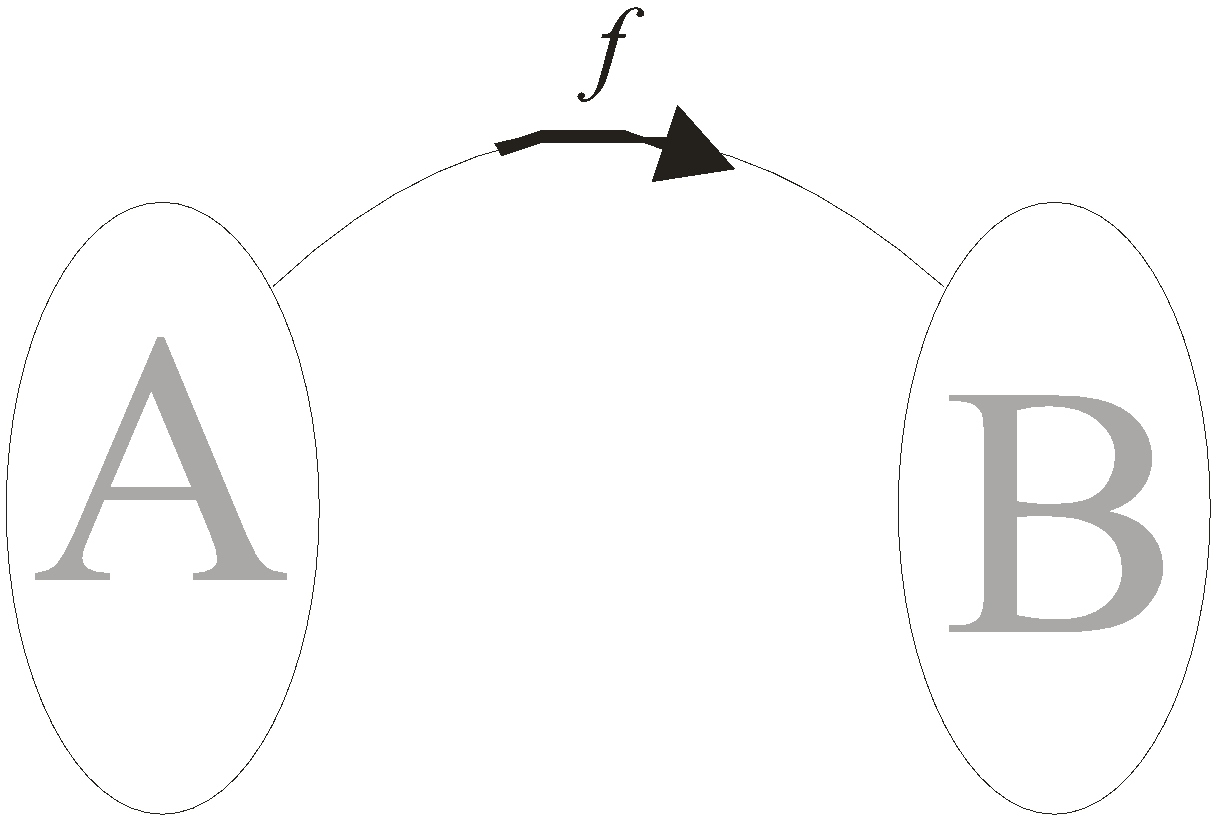Now, the basic terms: value, domain, codomain and range of function f are defined as follows:

Value of Function

If a is any element in A, such that aA, then

b = f(a)

is the value of f at x = a, where, bB

Domain of Function

The set of values of x (independent variable) can take so that the function is well defined is known as the domain of the function. For the arrangement assumed above, the set A is the domain of f.

Example: Let f : {a b c}is a function, that the domain of f is {a b c}.

Codomain of Function

The set of all possible outcomes for the function f i.e.set B is called the co-domain of the function f.

Example: Let f: {a, b, c}is a function, that the co-domain of f is.

Range of Function

The set of exact outcomes corresponding to the given domain of the function is called the range of that function.In oter words, the set of values of y (dependent variable) can take for all x belonging to its domain is called the range of the function.

Hence, the set {f(x)|xA, f(x)B} is the range of the function f.